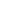true

# Free CBSE Class 9 Mathematics Unit 4-Geometry Construction Worksheets

Download free printable Construction Worksheets to practice. With thousands of questions available, you can generate as many Construction Worksheets as you want.

## Sample CBSE Class 9 Mathematics Unit 4-Geometry Construction Worksheet Questions

1.

State True/False:  To construct a triangle given its base, a base angle and the sum of the other two sides is required.

1. True 2. False
2.

State True/False:  To construct a triangle given its base, a base angle and the sum of the other two sides is required.

1. True 2. False
3.

Construct a right triangle whose base is 12cm and sum of its hypotenuse and other side is 18 cm.

4.

Construct an angle bisector of an angle of

5.

Construct a perpendicular bisector of line segment AB of length 7cm.

6.

Construct a triangle ABC in which AB = 5cm, BC = 7 cm, .

7.

Construct a triangle ABC in which BC = 6 cm, and .

8.

Construct a triangle whose sides are 4.6 cm, 3.0 cm and 6.1 cm.

9.

Construct a triangle ABC in which BC = 6 cm, and AB – AC = 3.5 cm.

10.

Draw an angle of 85° with the help of a protractor and bisect it.

Worksheets by UrbanPro

Our worksheets are designed to help students explore various topics, practice skills and enrich their subject knowledge, to improve their academic performance. Designed by Experts who have extensive experience and expertise in teaching a subject, these worksheets will improve your child's problem-solving skills and subject knowledge in a fun and interactive manner.
Check out our free customized worksheets across school boards, grades, subjects and levels of subject knowledge. You can download, print and share these worksheets with anyone, anywhere, anytime!

Get a custom worksheet to practice!

Select your topic & see the magic.

subjectSelect Chapter(s)

Chapters & Subtopics